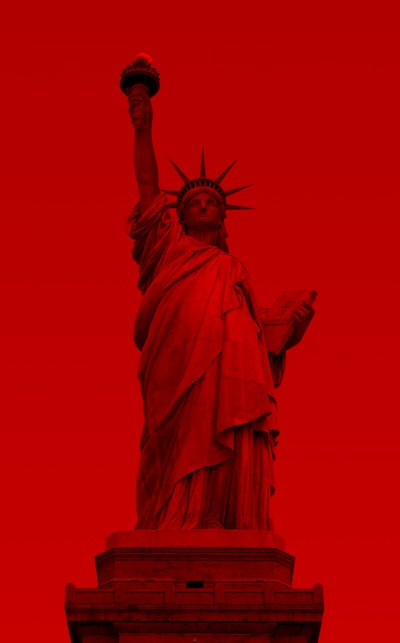# Image-6 Grayscale

In this section, we'll look at the structure of grayscale vs. color images, and some code to play with that difference.

## Gray Among The RGB - You Try It

• Demo experiment - visit the RGB explorer
• Figure out how to make a shade of gray
• e.g. RGB values to make: dark gray, medium gray, light gray
• We'll say that these grays lack "hue"
• Bonus: how do you make darker or lighter grays?

### Examples of gray colors in RGB:

red green blue color
50 50 50 dark gray
120 120 120 medium gray
200 200 200 light gray
250 200 200 not gray, reddish
0 0 0 black (a sort of gray)
255 255 255 white (ditto)

## Red Liberty Example ProblemHere is an image of the Statue of Liberty where all of the data is in the red values, so the whole image looks red (we saw this sort of image in an earlier puzzle solution). The green and blue values are all zero. This image looks quite wrong.

For this example, we'll write code to fix this image by copying the red value over to be used as the green and blue value. So for a pixel, if red is 27, set green and blue to also be 27. What is the code to do that? What will be the visual result of this?

• All green and blue values are 0
• The red values are real
• Here's what individual pixels look like:
red green blue color
65 0 0
113 0 0
204 0 0
19 0 0
... 0 0
• Change the image to be grayscale, not just red
• Change the red/green/blue values to be all equal for each pixel.
• What change to the pixels will accomplish this?
• Algorithm: for each pixel, set the green value to be the same as the red value
• Likewise, set the blue value to be the same as the red value
• Code: you try it below

image-gray1

Solution code:

## Converting Color To Grayscale• How to covert a regular color image to grayscale?
• Problem: for each pixel, how dark/light is it (ignoring hue)
• Choose a few pixels out of flowers.jpg, each in a row below
• Q: How to decide which pixel below is brightest? darkest?
red green blue color
pixel-1 250 25 25
pixel-2 0 75 75
pixel-3 150 225 225

Looking at just red or blue or green in isolation, it's hard to tell which pixel is brightest or darkest in the above table. The average combines and summarizes the three values into one number 0..255. The average shows how bright the pixel is, ignoring hue: 0 = totally dark, 255=totally bright, with intermediate average values corresponding to intermediate brightnesses. More complicated brightness measures are possible, but average is simple and works fine for our purposes.

• We compute average of red/green/blue values for each pixel
• To average 3 numbers, add them up and divide by 3
• average = (red + green + blue)/3
• Average combines red/green/blue into one number
• The average measures how bright the pixel is 0..255
• Ignoring hue
red green blue average
average = (red + green + blue) / 3
pixel-1 250 25 25 100 (medium bright)
pixel-2 0 75 75 50 (darkest)
pixel-3 150 225 225 200 (brightest)

## Code To Compute Pixel Average

• Compute the average value of a pixel:
• Algorithm: add red+green+blue, then divide by 3
• Code below computes the average, stores it in a variable "avg"
• We'll use that line whenever we want to compute the average
```  avg = (pixel.getRed() + pixel.getGreen() + pixel.getBlue())/3;
```

## Grayscale Conversion Algorithm

For this example, we'll write code to change the flowers.jpg image to grayscale, using the "average" strategy: for each pixel, compute the average of its red/green/blue values. This average number represents the brightness of the pixel 0..255. Then set the red, green, and blue values of the pixel to be that average number. The result is a grayscale version of the original color image. Once its working with flowers.jpg, try it with poppy.jpg or oranges.jpg. (Solution code available below)

• Pixel's average is effectively a "brightness" number 0..255
• Summarizes the 3 red/green/blue numbers as one number
• To change a pixel to grayscale:
-Compute the pixel's average value, e.g. 75
-Set the pixel's red/green/blue values to be the average
-e.g. red/green/blue all set to 75
• Now the pixel is gray, red/green/blue all equal

image-gray2

## Grayscale Followup Questions

• Q1: What happens if we pixel.setBlue(avg * 1.2)?
- we get a blue tint, since blue is now bigger than the other two
• Q2: must the "avg = ... " line be inside the loop?
• Yes, it must be inside the loop
• The equals sign (=) only works when that line is run
• (Unlike traditional math meaning of =)
• Each pixel has different red/green/blue values
• We need to re-do the addition / divide-3 for each pixel

Solution code:

## Grayscale Summary

• When red/green/blue values are equal .. shade of gray
• Average combines red/green/blue into one number
• The average measures how bright the pixel is 0..255
• Convert pixel to grayscale: set red, green, and blue to be the average
• Standard code line to compute average within loop. We'll use this line often for later problems.
`avg = (pixel.getRed() + pixel.getGreen() + pixel.getBlue())/3;`